• 线性回归公式推导线性模型线性回归一元线性回归公式推导多元线性回归公式推导对数几率回归介绍对数几率回归公式推导 线性模型 线性回归 一元线性回归公式推导 多元线性回归公式推导 对数几率回归 介绍 对数几率回归...


线性回归公式推导
线性模型一、线性回归（一）一元线性回归1. 由最小二乘法得出损失函数2. 证明损失函数

E

(

w

,

b

)

E(w,b)

是关于

w

w

和

b

b

的凸函数3. 对损失函数求关于b和w的一阶偏导数4. 令一阶偏导数为0解出

w

w

和

b

b

（二）多元线性回归1. 由最小二乘法求出损失函数2. 证明损失函数

E

w

^

E_{\hat w}

是关于

w

^

\hat w

的凸函数3. 令一阶偏导数为0，解出

w

^

\hat w

二、对数几率回归（一）广义线性模型（二）对数几率回归公式推导1. 对数几率回归模型2. 极大似然估计方法

本内容依据周志华老师的《机器学习》（西瓜书）总结而来。初次发文，若有不当的地方，还请指正。

线性模型
给定有

d

d

个属性描述的示例

x

=

(

x

1

;

x

2

;

.

.

.

;

x

d

)

x=(x_1;x_2;...;x_d)

，其中

x

i

x_i

是

x

x

在第

i

i

个属性上的取值，线性模型（linear model）试图学得一个通过属性的线性组合来进行预测的函数，即

f

(

x

)

=

w

1

x

1

+

w

2

x

2

+

.

.

.

+

w

d

x

d

+

b

f(x)=w_1x_1+w_2x_2+...+w_dx_d+b

一般用向量的形式写成

f

(

x

)

=

w

T

x

+

b

f(x)=w^Tx+b

其中

w

=

（

w

1

;

w

2

;

.

.

.

;

w

d

）

w =（w_1;w_2;...;w_d）

w

w

和

b

b

学得之后，模型就得以确定。
线性模型形式简单、易于建模，但蕴含着机器学习中的一些重要的基本思想。许多功能更为强大的非线性模型（nonlinear model）可在线性模型的基础上通过引入层级结构或高维映射而得。
一、线性回归
（一）一元线性回归
在线性回归问题中，首先考虑输入属性的数目只有一个，即一元线性回归问题，试图学得

f

(

x

i

)

=

w

x

i

+

b

，

使

得

f

(

x

i

)

≃

y

i

f(x_i)=wx_i+b，使得f(x_i)\simeq y_i

求解步骤： 1. 由最小二乘法得出损失函数E(w,b) 2. 证明损失函数E(w,b)是关于w和b的凸函数 3. 对损失函数求关于b和w的一阶偏导数 4. 令一阶偏导数等于0，解出w和b
1. 由最小二乘法得出损失函数

E

(

w

,

b

)

=

∑

i

=

1

m

(

y

i

−

f

(

x

i

)

)

2

=

∑

i

=

1

m

(

y

i

−

w

x

i

−

b

)

2

\begin{aligned}E_{(w,b)}&=\sum^m_{i=1}(y_i-f(x_i))^2\\ &=\sum_{i=1}^m (y_i-wx_i-b)^2\end{aligned}

均方误差有非常好的几何意义，它对应了常用的欧几里得距离或简称“欧氏距离”（Euclidean distance）。基于均方误差最小化来进行模型求解的方法称为“最小二乘法”（least square method）。在线性回归中，最小二乘法就是试图找到一条直线，使所有样本到直线上的欧氏距离之和最小。即求解目的为：

(

w

,

b

)

=

arg

⁡

min

⁡

(

w

,

b

)

∑

i

=

1

m

(

y

i

−

w

x

i

−

b

)

2

(w,b)=\mathop{\arg\min}\limits_{(w,b)}\sum^m_{i=1}(y_i-wx_i-b)^2

2. 证明损失函数

E

(

w

,

b

)

E(w,b)

是关于

w

w

和

b

b

的凸函数
二元函数判断凹凸性： 设

f

(

x

,

y

)

f(x,y)

在区域D上具有二阶连续偏导数，记

A

=

f

x

x

′

′

(

x

,

y

)

,

B

=

f

x

y

′

′

(

x

,

y

)

,

C

=

f

y

y

′

′

(

x

,

y

)

A=f^{''}_{xx}(x,y), B=f^{''}_{xy}(x,y), C=f^{''}_{yy}(x,y)

(1) 在D上恒有A>0，且

A

C

−

B

2

>

=

0

AC-B^2>=0

时，

f

(

x

,

y

)

f(x,y)

在区域D上是凸函数； (2) 在D上恒有A<0，且

A

C

−

B

2

>

=

0

AC-B^2>=0

时，

f

(

x

,

y

)

f(x,y)

在区域D上是凹函数。
二元凹凸函数求最值： 设

f

(

x

,

y

)

f(x,y)

是在开区域D内具有连续偏导数的凸（或者凹）函数

(

x

0

,

y

0

)

∈

D

(x_0,y_0)\in D

，且

f

x

′

(

x

0

,

y

0

)

=

0

,

f

y

′

(

x

0

,

y

0

)

=

0

f^{'}_{x}(x_0,y_0)=0,f^{'}_{y}(x_0,y_0)=0

，则

f

(

x

0

,

y

0

)

f(x_0,y_0)

必为

f

(

x

,

y

)

f(x,y)

在D内的最小值（或最大值）。
求

A

=

f

x

x

′

′

(

x

,

y

)

A=f^{''}_{xx}(x,y)

：

∂

E

(

w

,

b

)

∂

w

=

∂

∂

w

[

∑

i

=

1

m

(

y

i

−

w

x

i

−

b

)

2

]

=

2

∑

i

=

1

m

x

i

(

b

+

w

x

i

−

y

i

)

∂

2

E

(

w

,

b

)

∂

w

2

=

∂

∂

w

(

∂

E

(

w

,

b

)

∂

w

)

=

2

∑

i

=

1

m

x

i

2

\begin{aligned}{\partial E_{(w,b)}\over\partial w}&={\partial\over \partial w}[\sum ^m_{i=1}(y_i-wx_i-b)^2]\\ &=2\sum_{i=1}^mx_i(b+wx_i-y_i)\\ {\partial ^2E_{(w,b)}\over\partial w^2}&={\partial \over\partial w}({\partial{E_{(w,b)}}\over\partial w})\\&=2\sum ^m_{i=1}x_i^2 \end{aligned}

此即为

A

=

f

x

x

′

′

(

x

,

y

)

A=f^{''}_{xx}(x,y)

。
求

B

=

f

x

y

′

′

(

x

,

y

)

B=f^{''}_{xy}(x,y)

：

∂

2

E

(

w

,

b

)

∂

w

∂

b

=

∂

∂

b

(

∂

E

(

w

,

b

)

∂

w

)

=

2

∑

i

=

1

m

x

i

\begin{aligned} {\partial ^2E_{(w,b)}\over{\partial w\partial b}}&={\partial \over\partial b}({\partial E_{(w,b)}\over\partial w})\\ &=2\sum ^m_{i=1}x_i \end{aligned}

此即为

B

=

f

x

y

′

′

(

x

,

y

)

B=f^{''}_{xy}(x,y)

。
求

C

=

f

y

y

′

′

(

x

,

y

)

C=f^{''}_{yy}(x,y)

:

∂

E

(

w

,

b

)

∂

b

=

∂

∂

b

[

∑

i

=

1

m

(

y

i

−

w

x

i

−

b

)

2

]

=

2

(

m

b

−

∑

i

=

1

m

(

y

i

−

w

x

i

)

)

∂

2

E

(

w

,

b

)

∂

b

2

=

∂

∂

b

(

∂

E

(

w

,

b

)

∂

b

)

=

2

m

\begin{aligned} {\partial E_{(w,b)}\over{\partial b}}&={\partial \over \partial b}[\sum_{i=1}^m(y_i-wx_i-b)^2]\\ &=2(mb-\sum^m_{i=1}(y_i-wx_i))\\ {\partial ^2E_{(w,b)}\over{\partial b^2}}&={\partial \over \partial b}({\partial{E_{(w,b)}}\over\partial b})\\ &=2m \end{aligned}

此即为

C

=

f

y

y

′

′

(

x

,

y

)

C=f^{''}_{yy}(x,y)

。
易知

A

>

0

，

A

C

−

B

2

=

4

m

∑

i

=

1

m

(

x

i

−

x

‾

)

2

>

=

0

A>0，AC-B^2=4m\sum_{i=1}^m(x_i-\overline x)^2>=0

，故损失函数

E

(

w

,

b

)

E(w,b)

是关于

w

w

和

b

b

的凸函数得证。
3. 对损失函数求关于b和w的一阶偏导数

∂

E

(

w

,

b

)

∂

b

=

2

(

m

b

−

∑

i

=

1

m

(

y

i

−

w

x

i

)

)

∂

E

(

w

,

b

)

∂

w

=

2

∑

i

=

1

m

x

i

(

b

+

w

x

i

−

y

i

)

\begin{aligned} {\partial E_{(w,b)}\over{\partial b}}&=2(mb-\sum^m_{i=1}(y_i-wx_i))\\ {\partial E_{(w,b)}\over\partial w} &=2\sum_{i=1}^mx_i(b+wx_i-y_i) \end{aligned}

4. 令一阶偏导数为0解出

w

w

和

b

b

{

∂

E

(

w

,

b

)

∂

b

=

2

[

m

b

−

∑

i

=

1

m

(

y

i

−

w

x

i

)

]

=

0

∂

E

(

w

,

b

)

∂

w

=

2

∑

i

=

1

m

x

i

(

b

+

w

x

i

−

y

i

)

=

0

\begin{cases} {\partial E_{(w,b)}\over{\partial b}}&=2[mb-\sum^m_{i=1}(y_i-wx_i)]=0\\ {\partial E_{(w,b)}\over\partial w}&=2\sum_{i=1}^mx_i(b+wx_i-y_i)=0 \end{cases}

得：

b

=

1

m

∑

i

=

1

m

(

y

i

=

w

x

i

)

=

1

m

∑

i

=

1

m

y

i

−

w

⋅

1

m

∑

i

=

1

m

x

i

=

y

‾

−

w

x

‾

w

=

∑

i

=

1

m

y

i

x

i

−

y

‾

∑

i

=

1

m

x

i

∑

i

=

1

m

x

i

2

−

x

‾

∑

i

=

1

m

x

i

\begin{aligned} b&={1\over m}\sum^m_{i=1}(y_i=wx_i)\\ &={1\over m}\sum_{i=1}^my_i-w\cdot{1\over m}\sum_{i=1}^mx_i\\ &=\overline y-w\overline x\\ w=&{{\sum^m_{i=1}y_ix_i-\overline y\sum_{i=1}^mx_i}\over{\sum_{i=1}^mx_i^2-\overline x\sum_{i=1}^mx_i}} \end{aligned}

至此，便求出了一元线性模型的参数

w

w

和

b

b

。
（二）多元线性回归
由一元的情况扩展开来，此时样本由d个属性描述，此时我们试图学得

f

(

x

i

)

=

w

T

x

i

+

b

，

使

得

f

(

x

i

)

≃

y

i

f(x_i)=w^Tx_i+b，使得f(x_i)\simeq y_i

即多元线性回归问题，求解步骤与一元线性回归模型相似。
1. 由最小二乘法求出损失函数

f

(

x

i

)

=

w

T

x

i

+

b

=

(

w

1

,

w

2

,

.

.

.

,

w

d

)

(

x

i

1

x

i

2

.

.

.

x

i

d

)

+

b

=

b

记

作

w

d

+

1

(

w

1

,

w

2

,

.

.

.

,

w

d

,

w

d

+

1

)

(

x

i

1

x

i

2

⋮

x

i

d

1

)

=

w

^

T

x

^

i

\begin{aligned} f(x_i)&=w^Tx_i+b\\ &=(w_1,w_2,...,w_d) \begin{pmatrix} x_{i1}\\x_{i2}\\...\\x_{id} \end{pmatrix}+b\\ &\overset{b记作w_{d+1}}{=}(w_1,w_2,...,w_d,w_{d+1}) \begin{pmatrix} x_{i1}\\x_{i2}\\\vdots\\x_{id}\\1 \end{pmatrix}\\ &=\hat w^T\hat x_i \end{aligned}

如此一来，就将回归模型记为

f

(

x

^

i

)

=

w

^

T

x

^

i

f(\hat x_i)=\hat w^T\hat x_i

，便于后续的分析。接下来便由最小二乘法定义损失函数：

E

w

^

=

∑

i

=

1

m

(

y

i

−

f

(

x

^

i

)

)

=

∑

i

=

1

m

(

y

i

−

w

^

T

x

^

i

)

2

\begin{aligned} E_{\hat w}&=\sum_{i=1}^m(y_i-f(\hat x_i))\\ &=\sum_{i=1}^m(y_i-\hat w^T\hat x_i)^2 \end{aligned}

为便于分析，可做如下定义：

X

=

(

x

11

x

12

…

x

1

d

1

x

21

x

22

…

x

2

d

1

⋮

⋮

⋱

⋮

⋮

x

m

1

x

m

2

…

x

m

d

1

)

=

(

x

1

T

1

x

2

T

1

⋮

⋮

x

m

T

1

)

=

(

x

^

1

T

x

^

2

T

⋮

x

^

m

T

)

y

=

(

y

1

,

y

2

,

…

,

y

m

)

\begin{aligned} X&=\begin{pmatrix} x_{11}&x_{12}&\dots&x_{1d}&1\\ x_{21}&x_{22}&\dots&x_{2d}&1\\ \vdots&\vdots&\ddots&\vdots&\vdots\\ x_{m1}&x_{m2}&\dots&x_{md}&1 \end{pmatrix}=\begin{pmatrix} x_1^T&1\\ x_2^T&1\\ \vdots&\vdots\\ x_m^T&1 \end{pmatrix}=\begin{pmatrix} \hat x^T_1\\\hat x_2^T\\\vdots\\\hat x_m^T\end{pmatrix}\\\\ y&=(y_1,y_2,\dots,y_m) \end{aligned}

如此一来，可得：

(

y

1

−

w

^

T

x

^

1

y

2

−

w

^

T

x

^

2

⋮

y

m

−

w

^

T

x

^

m

)

=

(

y

1

y

2

⋮

y

m

)

−

(

w

^

T

x

^

1

w

^

T

x

^

2

⋮

w

^

T

x

^

m

)

=

(

y

1

y

2

⋮

y

m

)

−

(

x

^

1

T

w

^

x

^

2

T

w

^

⋮

x

^

m

T

w

^

)

=

y

−

X

w

^

\begin{aligned} \begin{pmatrix} y_1-\hat w^T\hat x_1\\ y_2-\hat w^T\hat x_2\\ \vdots\\ y_m-\hat w^T\hat x_m \end{pmatrix} &=\begin{pmatrix} y_1\\y_2\\\vdots\\y_m \end{pmatrix}-\begin{pmatrix} \hat w^T\hat x_1\\ \hat w^T\hat x_2\\ \vdots\\ \hat w^T\hat x_m \end{pmatrix}\\ &=\begin{pmatrix} y_1\\y_2\\\vdots\\y_m \end{pmatrix}-\begin{pmatrix} \hat x_1^T\hat w\\ \hat x_2^T\hat w\\ \vdots\\ \hat x_m^T\hat w \end{pmatrix}\\ &=y-X\hat w \end{aligned}

故：

E

w

^

=

(

y

−

X

w

^

)

T

(

y

−

X

w

^

)

E_{\hat w}=(y-X\hat w)^T(y-X\hat w)

2. 证明损失函数

E

w

^

E_{\hat w}

是关于

w

^

\hat w

的凸函数

∂

E

w

^

∂

w

^

=

∂

∂

w

^

[

(

y

−

X

w

^

)

T

(

y

−

X

w

^

)

]

=

∂

∂

w

^

(

y

T

−

w

^

T

X

T

)

(

y

−

X

w

^

)

=

∂

∂

w

^

(

y

T

y

−

w

^

T

X

T

y

−

y

T

X

w

^

+

w

^

T

X

T

X

w

^

)

=

∂

∂

w

^

(

−

w

^

T

X

T

y

−

y

T

X

w

^

+

w

^

T

X

T

X

w

^

)

=

(

−

y

T

X

)

T

−

X

T

y

+

(

X

T

X

+

X

T

X

)

w

^

=

2

X

T

(

X

w

^

−

y

)

\begin{aligned} {\partial E_{\hat w}\over \partial\hat w} &={\partial \over {\partial \hat w}}[(y-X\hat w)^T(y-X\hat w)]\\ &={\partial \over {\partial \hat w}}(y^T-\hat w^TX^T)(y-X\hat w)\\ &={\partial \over\partial \hat w}(y^Ty-\hat w^TX^Ty-y^TX\hat w+\hat w^TX^TX\hat w)\\ &={\partial \over\partial \hat w}(-\hat w^TX^Ty-y^TX\hat w+\hat w^TX^TX\hat w)\\ &=(-y^TX)^T-X^Ty+(X^TX+X^TX)\hat w\\ &=2X^T(X\hat w-y) \end{aligned}

上述式子中用到了矩阵微分公式：

∂

x

T

a

∂

x

=

∂

a

T

x

∂

x

=

a

,

∂

x

T

B

x

∂

x

=

(

B

+

B

T

)

x

{\partial x^Ta \over \partial x}={\partial a^Tx\over\partial x}=a, {\partial x^TBx\over \partial x}=(B+B^T)x

继续对

E

w

^

E_{\hat w}

求二阶导：

∂

2

E

w

^

∂

w

^

2

=

∂

∂

w

^

[

2

X

T

(

X

w

^

−

y

)

]

=

(

2

X

T

X

)

T

=

2

X

T

X

\begin{aligned} {\partial^2E_{\hat w}\over\partial\hat w^2}&={\partial\over\partial \hat w}[2X^T(X\hat w-y)]\\ &=(2X^TX)^T\\ &=2X^TX \end{aligned}

由多元实值函数凹凸性判定定理，若

X

T

X

X^TX

是正定矩阵，则

E

w

^

E_{\hat w}

为凸函数。
3. 令一阶偏导数为0，解出

w

^

\hat w

2

X

T

(

X

w

^

−

y

)

=

0

得

w

^

=

(

X

T

X

)

−

1

X

T

y

2X^T(X\hat w-y)=0\\得\hat w=(X^TX)^{-1}X^Ty

至此，便解出了多元线性回归模型。
二、对数几率回归
（一）广义线性模型
线性模型虽简单，却有丰富的变化，线性回归模型可简写为

y

=

w

T

x

+

b

y=w^Tx+b

，假设某一情况中对应的输出是在指数尺度上变化，那就可将输出的对数作为线性模型逼近的目标，即：

l

n

y

=

w

T

x

+

b

lny=w^Tx+b

上式在形式上仍是线性回归，但实质上已是在求取输入空间到输出空间的非线性函数映射。这里的对数函数起到了将线性回归模型的预测值与真实的标记联系起来的作用。
更一般地，考虑单调可微函数

g

(

⋅

)

g(\cdot)

，令

y

=

g

−

1

(

w

T

x

+

b

)

y=g^{-1}(w^Tx+b)

这样得到的模型称为“广义线性模型”（generalized linear model），其中函数

g

(

⋅

)

g(\cdot)

（二）对数几率回归公式推导
1. 对数几率回归模型
上一节讨论了如何使用线性回归模型进行学习，但若要做的是分类任务该怎么办？答案蕴含在广义线性模型中：只需找到一个单调可微函数将分类任务的真实标记与线性回归模型的预测值联系起来。
考虑二分类任务，其输出标记

y

∈

{

0

,

1

}

y\in\{0,1\}

，而线性回归模型产生的预测值

y

=

(

w

T

x

+

b

)

y=(w^Tx+b)

是实值，于是需要找到一个函数将实值转化为0/1值，对数几率函数就是这样一个合适的函数。对数几率函数如图所示：其表达式为：

y

=

1

1

+

e

−

z

y={1\over {1+e^{-z}}}

，将其作为

g

−

(

⋅

)

g^-(\cdot)

带入

y

=

g

−

1

(

w

T

x

+

b

)

y=g^{-1}(w^Tx+b)

，即可得对数几率回归模型：

y

=

1

1

+

e

−

(

w

T

x

+

b

)

=

e

w

T

x

+

b

1

+

e

w

T

x

+

b

y={1\over{1+e^{-(w^Tx+b)}}}={e^{w^Tx+b}\over1+e^{w^Tx+b}}

2. 极大似然估计方法
哪一组参数使得现在的样本观测值出现的概率最大，哪一组参数可能就是真正的参数，我们就用它作为参数的估计值，这就是所谓的极大似然估计。已知随机变量的

y

y

取1和0的概率分别为

p

(

y

=

1

∣

x

)

=

e

w

T

x

+

b

1

+

e

w

T

x

+

b

p

(

y

=

0

∣

x

)

=

1

1

+

e

w

T

x

+

b

p(y=1|x)={e^{w^Tx+b}\over1+e^{w^Tx+b}}\\p(y=0|x)={1\over1+e^{w^Tx+b}}

令

β

=

(

w

;

b

)

,

x

^

=

(

x

,

1

)

\beta=(w;b),\hat x=(x,1)

，则

w

T

x

+

b

w^Tx+b

可简写为

β

T

x

^

\beta^T\hat x

，于是上式可简化为

p

(

y

=

1

∣

x

)

=

e

β

T

x

^

1

+

e

β

T

x

^

=

p

1

(

x

^

;

β

)

p

(

y

=

0

∣

x

)

=

1

1

+

e

β

T

x

^

=

p

0

(

x

^

;

β

)

p(y=1|x)={e^{\beta^T\hat x}\over1+e^{\beta^T\hat x}}=p_1(\hat x;\beta)\\p(y=0|x)={1\over1+e^{\beta^T\hat x}}=p_0(\hat x;\beta)

可得：

p

(

y

∣

x

;

w

,

b

)

=

y

⋅

p

1

(

x

^

;

β

)

+

(

1

−

y

)

⋅

p

0

(

x

^

;

β

)

p(y|x;w,b)=y\cdot p_1(\hat x;\beta)+(1-y)\cdot p_0(\hat x;\beta)

或者：

p

(

y

∣

x

;

w

,

b

)

=

[

p

1

(

x

^

;

β

)

]

y

[

p

0

(

x

^

;

β

)

]

1

−

y

p(y|x;w,b)=[p_1(\hat x;\beta)]^y[p_0(\hat x;\beta)]^{1-y}

在许多情况下，求解似然函数的对数

l

n

L

(

w

)

lnL(w)

比求解似然函数本身

L

(

w

)

L(w)

更简单，通常称

l

n

L

(

w

)

lnL(w)

为对数似然函数。

l

(

w

,

b

)

=

∑

i

=

1

m

l

n

p

(

y

i

∣

x

i

;

w

,

b

)

l(w,b)=\sum_{i=1}^mlnp(y_i|x_i;w,b)

将概率值带入对数似然函数，最大化似然函数，等价于最小化：

l

(

β

)

=

∑

r

=

1

m

(

−

y

i

β

T

x

^

i

+

l

n

(

1

+

e

β

T

x

^

i

)

)

\begin{aligned} l(\beta)&=\sum_{r=1}^m(-y_i\beta^T\hat x_i+ln(1+e^{\beta^T\hat x_i}))\end{aligned}

便可求出参数的估计：

β

∗

=

a

r

g

min

⁡

β

l

(

β

)

\begin{aligned}\beta^*&=arg\,\min_{\beta}l(\beta) \end{aligned}


展开全文机器学习
• 机器学习-线性回归线性回归背景最小二乘法计算线性回归数据的准备把数据转换成数学损失函数根据损失函数计算最终结果总结 最近老师让我学习线性回归编程模型，此篇介绍使用最小二乘法实现单机器线性回归线性回归...


机器学习-线性回归
线性回归背景最小二乘法计算线性回归数据的准备把数据转换成数学损失函数根据损失函数计算最终结果
总结

最近老师让我学习线性回归编程模型，此篇介绍使用最小二乘法实现单机器线性回归。

线性回归背景
在生活中，我们常常会发现，一个现象可以由很多因素共同作用才会发生，我们把现象称为t,把影响它的因素1,因素2,因素三…因素m称为x1,x2…xm，把每个因素的影响权重称为w1,w2…wm。那么会存在一个现象，即 t=w1x1+w2x2+…+wm*xm。例如房价，受到地方，人口，银行，土地政策四个因素的共同作用,我们以全国三个城市的房价为例，即我手上一共有三组数据：
城市省GDP人口流入量银行土地起拍房价石家庄3.6万亿40万4.35%80001.2万西安2.44万亿11万4.35%70001.3万天津1.88万亿0.78万4.35%1.5万3万
假如我手上哈尔滨的相关数据，我需要根据我已知数据找到一个房价预测公式，该公式包含上述因素和因素对应权重，即 房价=因素1权重1+因素2权重2+…+因素m*权重m。 最后，我通过哈尔滨的一系列因素值，能够较为准确的预测出哈尔滨的房价。以上通过样例数据找各个因素的权重的过程就是线性回归。
最小二乘法计算线性回归
数据的准备
因为我们要根据样例数据去预测未知结果，所以我们的样例数据格式为：
样例数据最终结果因素1权重因素2权重…因素m权重
我们使用t(i)去记录第i个样例数据最终结果。 我们使用(x(i,0),x(i,1),x(i,2)…x(i,m))表示第i组数据,一共n组数据。 令w(j)表示第j个因素的权重。
把数据转换成数学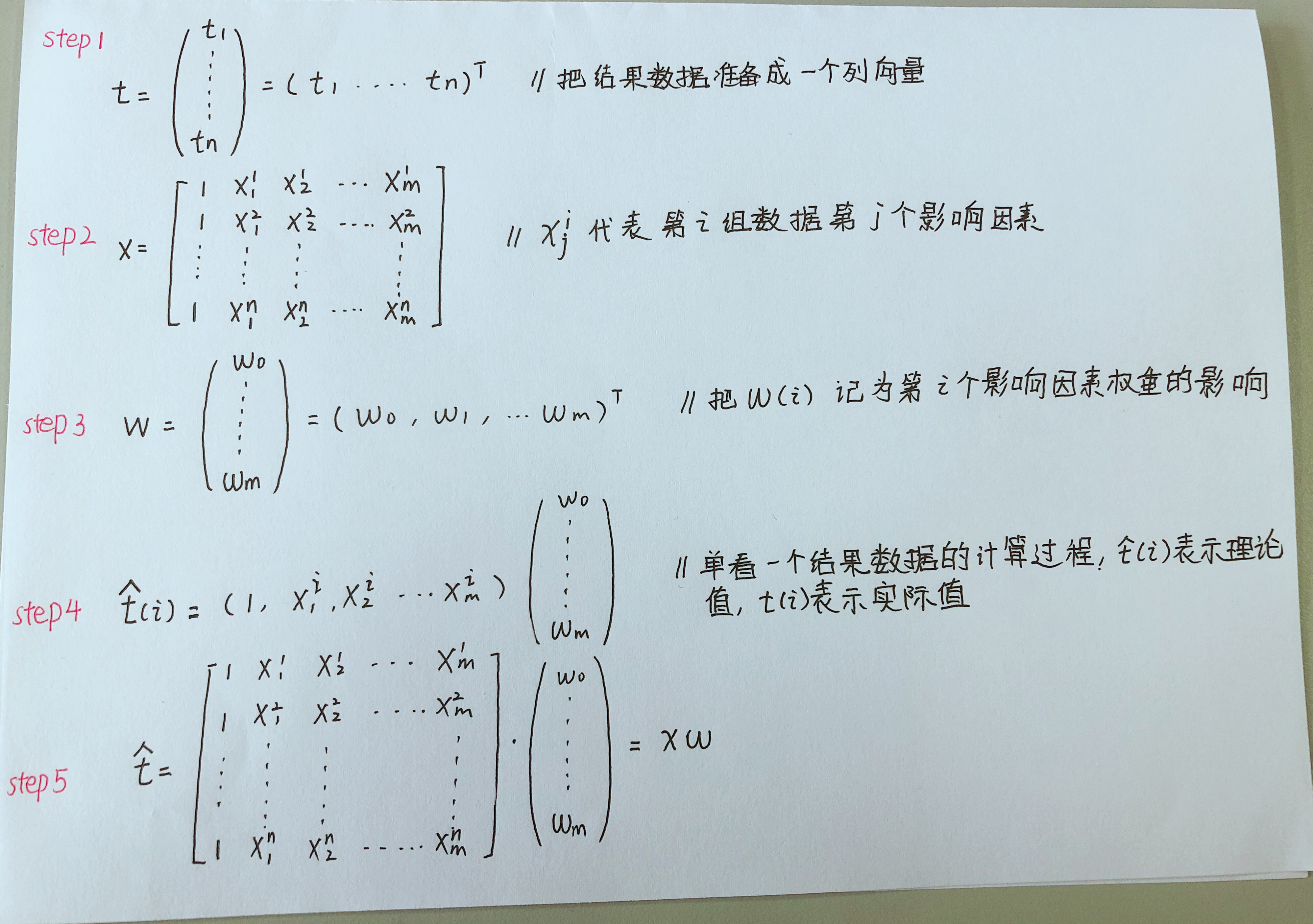损失函数
损失函数（loss function）或代价函数（cost function）是将随机事件或其有关随机变量的取值映射为非负实数以表示该随机事件的“风险”或“损失”的函数。这里我们只说平方损失函数：实际结果和观测结果之间差距的平方和。使用损失函数是一种能够查看当前模型准不准确的手段。损失函数越小，证明当前模型越准确。损失函数计算公式为：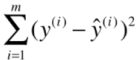损失函数在本例中的推导如下： 损失函数变形 step 1：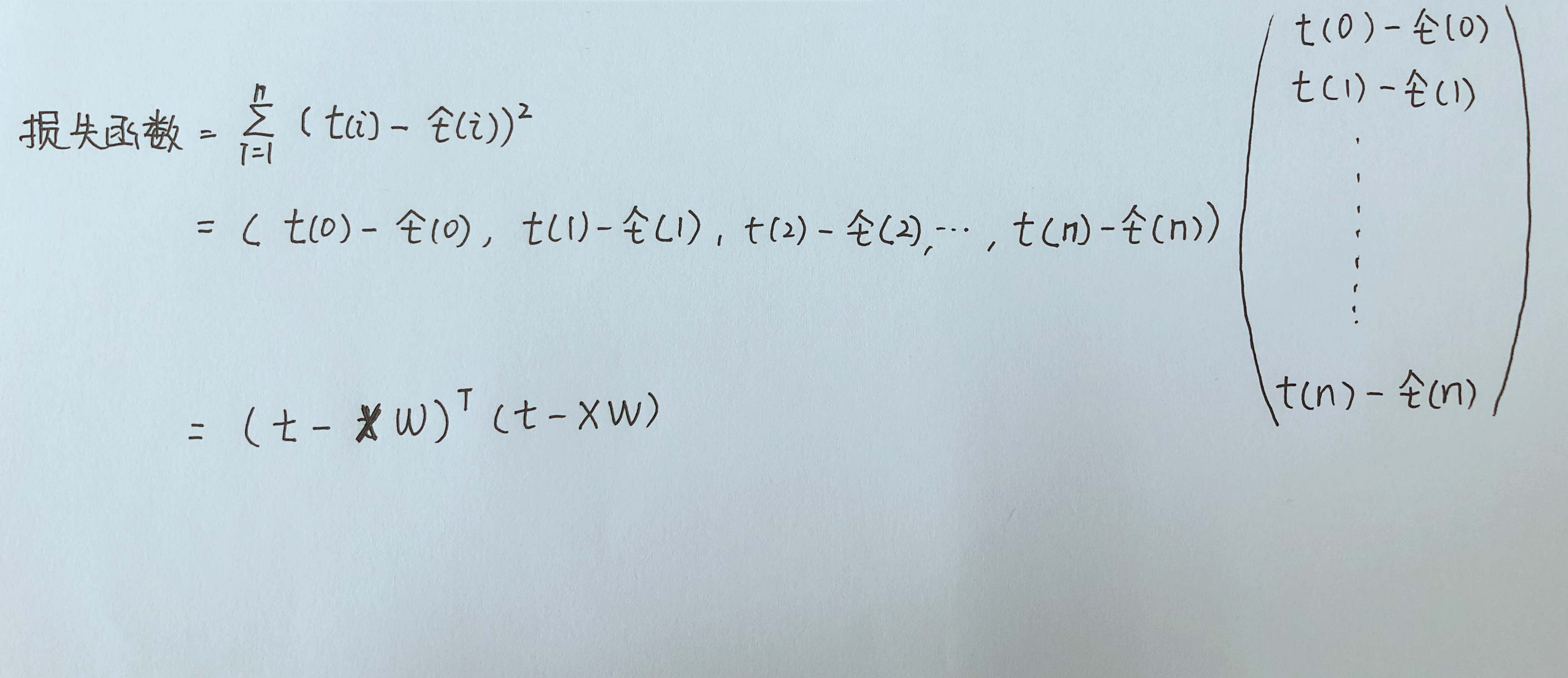损失函数变形 step 2: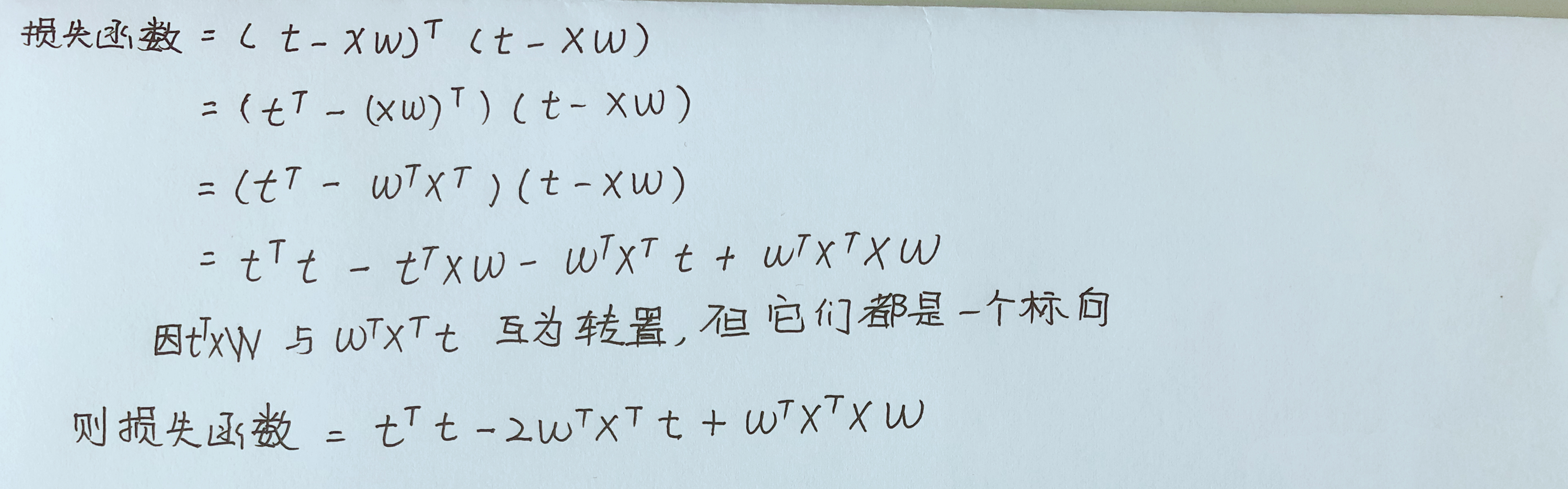根据损失函数计算最终结果
对于上述图片中的损失函数来说，t是我们的样例数据结果值，它是已知的，x是我们样例数据影响因素值，它们也是已知。事实上，对于该损失函数来说，它是一个多元函数，它的自变量为：w0,w1,w2…wm，若要使损失函数降到最小，实际上是要一组w值，使得该损失函数的值能够最小。 由此涉及到多元函数求导问题，导数为0的地方则为极值处。
由于我们把一组w当做一个列向量来看待，现在给出针对列向量的微分公式：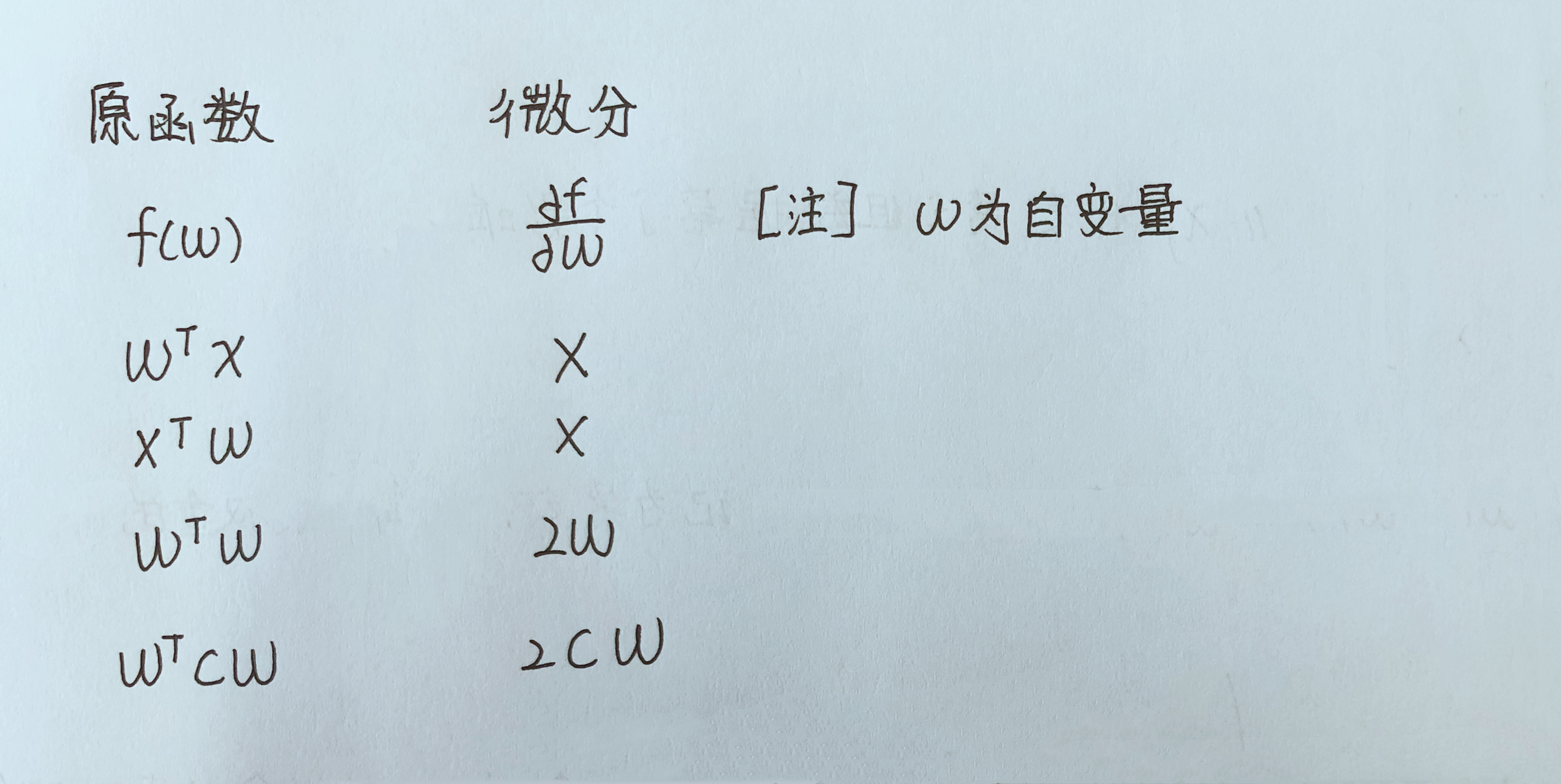根据上图公式，对损失函数求微分的过程如下：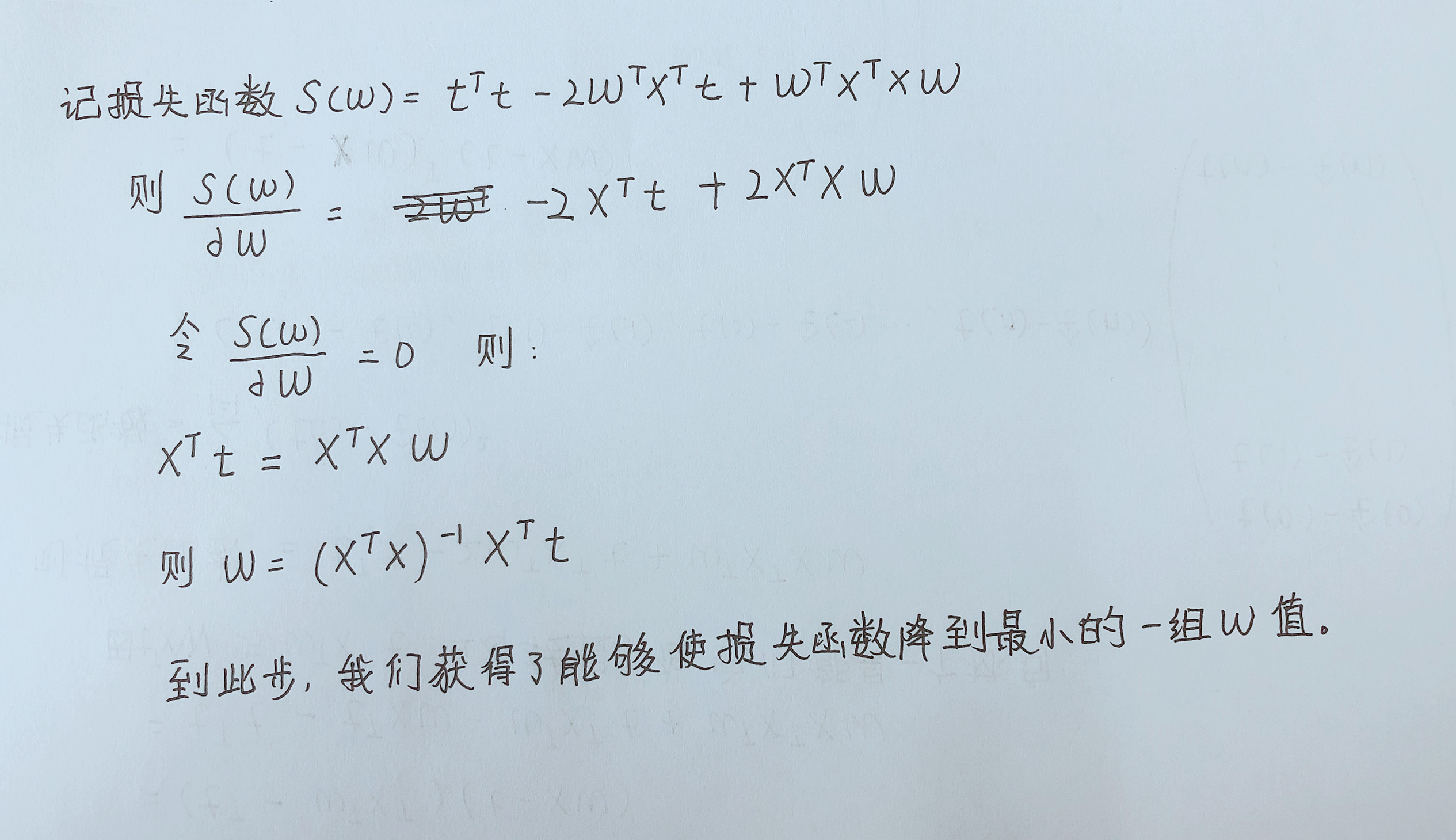总结
对此，线性回归最小二乘法的公式推导过程已经结束。但是，其中涉及的一些公式，我只是使用，并不知道它们的完整推导过程，其中这个问题最严重地体现在对一系列变量(列向量)的求微分的公式。我学习这个线性回归，主要是想用线性回归为例，学习GPU和CPU下处理大数据的性能对比。本着想写一个系列总结，有利于自己将来学习的想法，下一节打算写以一元线性回归为例的CUDA的最简单使用。
展开全文最小二乘法 机器学习
• 本篇一元回归主要参考周志华老师《机器学习》，主要工作是把周老师在书中省略的公式推导过程详细叙述清楚。 对于样本集 DDD = { (x1x1x_1,y1y1y_1), (x2x2x_2,y2y2y_2), …, (xmxmx_m,ymymy_m) } 线性回归模型就是...
此乃开博首篇，先用简单的问题来当做开胃菜！
本篇一元回归主要参考周志华老师《机器学习》，主要工作是把周老师在书中省略的公式推导过程详细叙述清楚。
对于样本集

D

D

$D$ = { (x1${x}_{1}$$x_1$,

y1

y

1

$y_1$), (

x2

x

2

$x_2$,

y2

y

2

$y_2$), …, (

xm

x

m

$x_m$,

ym

y

m

$y_m$) } 线性回归模型就是要找到一个模型

f(x)=wx+b

f

(

x

)

=

w

x

+

b

$f(x)=wx+b$ 使得

∀i∈[1,m]

∀

i

∈

[

1

,

m

]

$\forall i\in [1,m]$ 都有

f(xi)

f

(

x

i

)

$f(x_i)$ 尽可能地接近于

yi

y

i

$y_i$ 所以我们使每一个样本的预测值与真实值的差的平方和最小，即：

(w∗,b∗)=argw,bmin∑i=1m(f(xi)−yi)2=argw,bmin∑i=1m(wxi+b−yi)2(1)

(

w

∗

,

b

∗

)

=

arg

w

,

b

⁡

min

∑

i

=

1

m

(

f

(

x

i

)

−

y

i

)

2

(1)

=

arg

w

,

b

⁡

min

∑

i

=

1

m

(

w

x

i

+

b

−

y

i

)

2

\begin{align*}
(w^*, b^*)&=\arg_{w,b} \min\sum_{i=1}^m{(f(x_i)-y_i)}^2\\
&=\arg_{w,b} \min\sum_{i=1}^m{(wx_i+b-y_i)}^2\tag 1
\end{align*}
这可以看做是对多元函数

h(w,b)=∑mi=1(wxi+b−yi)2

h

(

w

,

b

)

=

∑

i

=

1

m

(

w

x

i

+

b

−

y

i

)

2

$h(w,b)=\sum_{i=1}^m{(wx_i+b-y_i)}^2$ 求最小值，则按照微积分的知识，函数

h

h

$h$ 对 w$w$$w$ 和

b

b

$b$ 分别求偏导，并令二者的偏导数为零，则此时对应的 w∗${w}^{\ast }$$w^*$ 和

b∗

b

∗

$b^*$ 便是使得

h

h

$h$ 取最小的值。故，求偏导后得到：
∂h(w,b)∂w=∑i=1m2(wxi+b−yi)∗xi=2(w∑i=1mx2i−∑i=1m(yi−b)xi)(2)$\begin{array}{rl}\frac{\mathrm{\partial }h\left(w,b\right)}{\mathrm{\partial }w}& =\sum _{i=1}^{m}2\left(w{x}_{i}+b-{y}_{i}\right)\ast {x}_{i}\\ \text{(2)}& & =2\left(w\sum _{i=1}^{m}{x}_{i}^{2}-\sum _{i=1}^{m}\left({y}_{i}-b\right){x}_{i}\right)\end{array}$\begin{align*}
\frac{\partial h(w,b)}{\partial w}&=\sum_{i=1}^m2(wx_i+b-y_i)*x_i\\
&=2\Biggl(w\sum_{i=1}^mx_i^2-\sum_{i=1}^m(y_i-b)x_i\Biggr)\tag 2
\end{align*}

∂h(w,b)∂b=∑i=1m2(wxi+b−yi)∗1=2(mb−∑i=1m(yi−wxi))(3)

∂

h

(

w

,

b

)

∂

b

=

∑

i

=

1

m

2

(

w

x

i

+

b

−

y

i

)

∗

1

(3)

=

2

(

m

b

−

∑

i

=

1

m

(

y

i

−

w

x

i

)

)

\begin{align*}
\frac{\partial h(w,b)}{\partial b}&=\sum_{i=1}^m2(wx_i+b-y_i)*1\\
&=2\Biggl(mb-\sum_{i=1}^m(y_i-wx_i)\Biggl)\tag 3
\end{align*}
分别令二者等于0便可以得到

w

w

$w$ 和 b$b$$b$ 的最优解：

wb=∑mi=1yi(xi−x¯)∑mi=1x2i−1m(∑mi=1xi)2=1m∑i=1m(yi−wxi)(4)(5)

(4)

w

=

∑

i

=

1

m

y

i

(

x

i

−

x

¯

)

∑

i

=

1

m

x

i

2

−

1

m

(

∑

i

=

1

m

x

i

)

2

(5)

b

=

1

m

∑

i

=

1

m

(

y

i

−

w

x

i

)

\begin{align*}
w&=\frac{\sum_{i=1}^my_i(x_i-\bar x)}{\sum_{i=1}^mx_i^2-\frac {1}{m}{ (\sum_{i=1}^mx_i)}^2}\tag 4\\
b&=\frac 1 m \sum_{i=1}^m(y_i-wx_i)\tag 5
\end{align*}
这其中，式(5)是很容易从式(3)得到的，此处不赘述，但是式(4)的得来并没有这么直观，我们来详细推导一下。

令式(2)等于 0 则有：

w∑i=1mx2i=∑i=1mxiyi−b∑i=1mxi(6)

(6)

w

∑

i

=

1

m

x

i

2

=

∑

i

=

1

m

x

i

y

i

−

b

∑

i

=

1

m

x

i

w\sum_{i=1}^mx_i^2=\sum_{i=1}^mx_iy_i-b\sum_{i=1}^mx_i\tag 6
将式(5)代入式(6)得：

w∑i=1mx2i=∑i=1mxiyi−1m∑i=1mxi(∑i=1m(yi−wxi))=∑i=1mxiyi−x¯(∑i=1myi)+1m∑i=1mxi∗∑i=1mwxi=∑i=1mxiyi−x¯∑i=1myi+wm(∑i=1mxi)2(7)

w

∑

i

=

1

m

x

i

2

=

∑

i

=

1

m

x

i

y

i

−

1

m

∑

i

=

1

m

x

i

(

∑

i

=

1

m

(

y

i

−

w

x

i

)

)

=

∑

i

=

1

m

x

i

y

i

−

x

¯

(

∑

i

=

1

m

y

i

)

+

1

m

∑

i

=

1

m

x

i

∗

∑

i

=

1

m

w

x

i

(7)

=

∑

i

=

1

m

x

i

y

i

−

x

¯

∑

i

=

1

m

y

i

+

w

m

(

∑

i

=

1

m

x

i

)

2

\begin{align*}
w\sum_{i=1}^mx_i^2&=\sum_{i=1}^mx_iy_i-\frac 1 m \sum_{i=1}^mx_i\Biggl( \sum_{i=1}^m(y_i-wx_i)\Biggr)\\
&=\sum_{i=1}^mx_iy_i-\bar x\Biggl( \sum_{i=1}^my_i\Biggr)+\frac 1 m\sum_{i=1}^mx_i*\sum_{i=1}^mwx_i\\
&=\sum_{i=1}^mx_iy_i-\bar x \sum_{i=1}^my_i+\frac w m\Biggl( \sum_{i=1}^mx_i\Biggr)^2\tag 7
\end{align*}
式(7)最右的平方项移项到左边可得：

w∑i=1mx2i−wm(∑i=1mxi)2=∑i=1mxiyi−x¯∑i=1myi

w

∑

i

=

1

m

x

i

2

−

w

m

(

∑

i

=

1

m

x

i

)

2

=

∑

i

=

1

m

x

i

y

i

−

x

¯

∑

i

=

1

m

y

i

w\sum_{i=1}^mx_i^2-\frac w m \Biggl( \sum_{i=1}^mx_i\Biggr)^2=\sum_{i=1}^mx_iy_i-\bar x \sum_{i=1}^my_i

w[∑i=1mx2i−1m(∑i=1mxi)2]=∑i=1mxiyi−x¯∑i=1myi(8)

(8)

w

[

∑

i

=

1

m

x

i

2

−

1

m

(

∑

i

=

1

m

x

i

)

2

]

=

∑

i

=

1

m

x

i

y

i

−

x

¯

∑

i

=

1

m

y

i

w\Biggl[\sum_{i=1}^mx_i^2-\frac 1 m \Biggl( \sum_{i=1}^mx_i\Biggr)^2\Biggl]=\sum_{i=1}^mx_iy_i-\bar x\sum_{i=1}^my_i\tag 8
式(8)变形后即可得式(4)，至此，

w

w

$w$ 和 b$b$$b$ 的表达式都只与观测样本有关，所以可以通过样本观测值来估计

w

w

$w$ 和 b$b$$b$ 。

下一篇博客将介绍多元线性回归，希望自己能坚持下去！！！
展开全文• 线性回归 (公式推导+python实现+应用举例+sklearn应用) 线性回归 (公式推导+python实现+应用举例+sklearn应用) 1.公式推导 2.用数据举例 * 简单介绍下归一化和标准化 3.python实现线性回归 4.scikit-learn中的...
线性回归 (公式推导+python实现+应用举例+sklearn应用)

文章目录
线性回归 (公式推导+python实现+应用举例+sklearn应用)1.公式推导2.用数据举例* 简单介绍下归一化和标准化
3.python实现线性回归4.scikit-learn中的线性模型5.总结及了解扩展内容

1.公式推导

线性模型形式如下:

h

θ

(

x

)

=

b

+

θ

1

x

1

+

θ

2

x

2

+

⋯

+

θ

n

x

n

=

b

+

∑

i

=

1

n

θ

i

x

i

h_\theta(x) =b+ \theta_1x_1 + \theta_2x_2 + \cdots + \theta_nx_n = b+\sum_{i=1}^{n}{\theta_i x_i}

写成向量形式即:

h

θ

(

x

)

=

θ

T

x

+

b

h_\theta(x) = \theta^Tx+b

将其转化为矩阵表达形式，即:

h

θ

(

X

)

=

X

θ

h_\theta(X) = X\theta

而我们的损失函数为:

J

(

θ

)

=

1

2

m

∑

i

=

1

m

(

h

θ

(

x

(

i

)

)

−

y

(

i

)

)

2

J(\theta) = \frac{1}{2m} \sum_{i=1}^{m}{(h_{\theta}(x^{(i)}) - y^{(i)}})^2

把均方误差(mean square error) 即 欧氏距离(Euclidean distance) 除以

2

m

2m

得到我们的 损失函数(cost functino): 注: 基于均方误差最小化来进行模型求解的方法叫做最小二乘法(least square method)

J

(

θ

)

=

1

2

m

(

X

θ

−

Y

)

T

(

X

θ

−

Y

)

=

1

2

m

(

θ

T

X

T

−

Y

T

)

(

X

θ

−

Y

)

=

1

2

m

(

θ

T

X

T

X

θ

−

θ

T

X

T

Y

−

Y

T

X

θ

+

Y

T

Y

)

J(\theta) = \frac{1}{2m} (X\theta - Y)^T (X\theta - Y) = \frac{1}{2m}(\theta^TX^T - Y^T)(X\theta - Y) = \frac{1}{2m}(\theta^TX^TX\theta - \theta^TX^TY - Y^TX\theta + Y^TY)

补充下矩阵求导法则:

d

A

B

d

B

=

A

T

\frac{\mathrm{d}AB }{\mathrm{d}B} = A^T

d

A

T

B

d

A

=

B

\frac{\mathrm{d}A^TB }{\mathrm{d}A} = B

d

X

T

A

X

d

X

=

2

A

X

\frac{\mathrm{d}X^TAX }{\mathrm{d}X} = 2AX

设我们有

m

m

个样本，

n

n

个属性，则

X

X

是

m

∗

(

n

+

1

)

m * (n+1)

矩阵 (第1列全为1), 而

θ

\theta

是

n

+

1

n+1

维向量, 对

J

(

θ

)

J(\theta)

求导:

∂

J

(

θ

)

∂

θ

=

1

2

m

(

2

X

T

X

θ

−

X

T

Y

−

X

T

Y

)

=

1

m

X

T

(

X

θ

−

Y

)

\frac{\partial J(\theta)}{\partial \theta} = \frac{1}{2m}(2X^TX\theta - X^TY - X^TY) = \frac{1}{m} X^T(X\theta - Y)

要求最小值，先令其为

0

0

，得到:

θ

=

(

X

T

X

)

−

1

X

T

Y

\theta = (X^TX)^{-1} X^TY

将

θ

\theta

代入线性模型得到:

h

θ

(

X

)

=

X

(

X

T

X

)

−

1

X

T

Y

h_\theta(X) = X(X^TX)^{-1} X^TY

这是我们直接得出的结果，但是有两点需要注意:
这是建立在

X

T

X

X^TX

可逆的基础上，若其不可逆则不能用该公式计算量实在是太大了,光是

(

X

T

X

)

−

1

(X^TX)^{-1}

就需要计算

(

n

+

1

)

∗

(

n

+

1

)

(n+1) * (n+1)

矩阵的逆
因为代价函数对

θ

j

\theta_j

求偏导得到:

∂

J

(

θ

)

∂

θ

j

=

1

m

∑

i

=

1

m

(

h

θ

(

x

i

)

−

y

i

)

x

j

\frac{\partial{J(\theta)}}{\partial{\theta_j}} = \frac{1}{m}\sum_{i=1}^{m}{(h_{\theta}(x_i) - y_i})x_j

则对

θ

\theta

的更新如下:
until convergence:{    for j in 0,1,2…n{

θ

j

:

=

θ

j

−

α

1

m

∑

i

=

1

m

(

h

θ

(

x

i

)

−

y

i

)

x

j

\theta_j := \theta_j - \alpha\frac{1}{m}\sum_{i=1}^{m}{(h_{\theta}(x_i) - y_i})x_j

} }
同样地，将其转化为矩阵形式 注:这里

α

\alpha

是学习速率,

θ

\theta

是

(

n

+

1

,

1

)

(n+1, 1)

的向量;

X

X

是

(

m

,

n

+

1

)

(m, n+1)

的矩阵;

Y

Y

是

(

m

,

1

)

(m, 1)

的向量,

h

θ

(

X

)

h_\theta(X)

也是

(

m

,

1

)

(m, 1)

的向量:

θ

=

θ

−

α

m

X

T

⋅

(

h

θ

(

X

)

−

Y

)

\theta = \theta - \frac{\alpha}{m}X^T\cdot(h_\theta(X) - Y)

注意

θ

\theta

是一个数，所以这里用的是点乘

2.用数据举例
这里导入的是高炉数据，高炉的含硅(Si)量预测可是个经典的问题 首先获取原始数据的DataFrame : data
import numpy as np
import matplotlib.pyplot as plt
import pandas as pd
# 这是我的环境需要，大多数人并不需要加以下两行代码
import sys
sys.path.append("C:\\python\\lib\\site-packages")
%matplotlib inline

print(data.describe())

得到结果:

SiScoalwindcount1000.0000001000.0000001000.0000001000.000000mean0.4594100.02378813.1594001759.686810std0.1109650.0097432.12165180.083738min0.2100000.0060000.120000833.52000025%0.3800000.01700012.4475001733.73250050%0.4400000.02300013.5450001775.24000075%0.5200000.03000014.3700001802.390000max1.2600000.07800017.7000001897.230000
可以看到，该数据集分为3个特征: S(含硫量), coal(喷煤量), wind(风量) 且一共有1000条数据，不存在数据缺失

* 简单介绍下归一化和标准化
现在我们需要对数据预处理，即归一化(Normalization) 或 标准化(Standardization)
什么是归一化 (归一化目标是啥)  1.把数据映射到(0, 1)之间 2.去量纲化  和标准化的区别是啥  标准化不会改变数据的分布,归一化会改变数据分布  为什么要做归一化  1.提升模型的收敛(convergence)速度 如果没有做归一化，各特征尺度不一样的话，会造成目标函数整个图形特别狭长，梯度下降的过程会走很多"弯路" 2.有助于提升模型的精度 这在牵涉到距离计算的算法如SVM时，提升效果非常显著, 因为如果这个特征变化特别大，距离计算就主要取决于这个特征，不符合实际情况  常用归一化算法:  线性转换:

y

=

x

−

min

⁡

max

⁡

−

min

⁡

y = \frac{x - \min}{\max - \min}

常用标准化算法:  1.MinMax规范化(线性变换):

y

=

x

−

min

⁡

max

⁡

−

min

⁡

∗

(

max

⁡

^

−

min

⁡

^

)

+

min

⁡

^

y = \frac{x-\min}{\max - \min} * (\hat{\max} - \hat{\min}) + \hat{\min}

注:

m

a

x

^

、

m

i

n

^

\hat{max} 、 \hat{min}

分别是新的最大值与最小值 2.Z-Score规范化(标准差标准化):

y

=

x

−

μ

σ

y = \frac{x - \mu}{\sigma}

注: 经过处理的数据符合标准正态分布，均值为0，标准差为1 3.对数Logistic规范化 :

y

=

1

1

+

e

−

x

y = \frac{1}{1 + e^{-x}}

4.log函数规范化 :

x

=

lg

⁡

x

lg

⁡

m

a

x

(

x

)

x = \frac{\lg{x}}{\lg{max(x)}}

注: 属于非线性的标准化，适用于数据分化大的场景，有些值特别大，有些值特别小
这里我们选择手动实现一个Z-Score的标准化:
def standard(X):
# np.array() 可将其它一些数据转化为numpy.ndarray类型的数据方便进行矩阵运算, 而np.ndarray()是将其它numpy.ndarray作为输入
X_norm = np.array(X)

# 创建一个1行，和X列宽等长的行向量
mu = np.zeros((1, X.shape))
sigma = np.zeros((1, X.shape))

mu = np.mean(X_norm, axis=0) # 求每一列的平均值，axis=0指定求的是列的均值
sigma = np.std(X_norm, axis=0)
for i in range(X.shape): # 遍历每一列
X_norm[: ,i] = (X_norm[: ,i] - mu[i]) / sigma[i]

return X_norm, mu, sigma

data_norm, mu, sigma = standard(data)
print(data_norm)

得到结果:
array([[ 0.18564659, -0.38900304, -0.48071521, -1.441423  ],
[ 0.36597354, -1.41593821, -0.72592996, -1.33435646],
[-0.35533425, -1.41593821, -1.33896682, -0.70095235],
...,
[-0.1750073 , -1.21055117, -0.45242121, -1.71614797],
[ 0.00531965,  0.22715806, -0.99943871, -0.60125679],
[-0.71598815, -0.90247062,  0.4954281 , -1.00253766]])

接下来我们data_norm再分割为Samples(样本矩阵)和Labels(结果向量)
Samples = data_norm[:, 1:]
print(Samples)

结果如下
array([[-0.38900304, -0.48071521, -1.441423  ],
[-1.41593821, -0.72592996, -1.33435646],
[-1.41593821, -1.33896682, -0.70095235],
...,
[-1.21055117, -0.45242121, -1.71614797],
[ 0.22715806, -0.99943871, -0.60125679],
[-0.90247062,  0.4954281 , -1.00253766]])

再检查标记向量:
Labels = data_norm[:, 0:1]
print(Labels.shape)

结果如下:
(1000, 1)

3.python实现线性回归
"""
代价函数
X是样本矩阵(m, n+1), y是结果向量(m, 1), theta是参数向量(n+1, 1)
返回一个数，即代价值
"""
def get_cost(X, y, theta):
# 对numpy.ndarray来说，np.dot()才是真正的线性代数中的矩阵相乘
return np.dot((np.transpose(np.dot(X,theta) - y)) , (np.dot(X,theta) - y)) / (2*y.shape)

"""
计算梯度下降, 返回最终学得的theta向量和cost function的变化list
X: (m, n+1)样本矩阵
y: (m, 1)结果向量
theta: (n+1, 1)参数向量
rate: 学习速率
rounds: 训练轮次
intercept: 若为True,则需要给样本矩阵X增加常数列
"""
def gradient_descent(X, y, rate, rounds, intercept=True):
iX = X.copy() # 如果不用copy()函数，改变iX同样会改变X
if intercept == True:
iX = np.column_stack((np.ones(iX.shape), iX)) # 给第一列全加上1

T = np.full(shape=(iX.shape, 1), fill_value=0.5, dtype=np.float)
L, costs = [], []

for i in range(rounds):
H = np.dot(iX, T) # 求内积，先计算出假设函数
T = T - (rate/y.shape) * np.dot(np.transpose(iX), (H-y))
L.append(i)
costs.append(get_cost(iX, y, T).tolist())

return T, costs

训练一个模型出来:
Theta, Costs = gradient_descent(Samples, Labels, 0.1, 40)

再绘出训练过程中代价函数变化曲线:
plt.plot(Costs)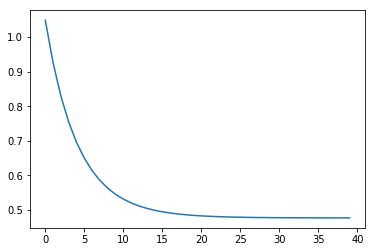4.scikit-learn中的线性模型
from sklearn import linear_model
from sklearn.preprocessing import StandardScaler

scaler = StandardScaler()
scaler.fit(data)

data_norm = scaler.transform(data)
print(data_norm)

查看scaler缩放后的数据:
array([[ 0.18564659, -0.38900304, -0.48071521, -1.441423  ],
[ 0.36597354, -1.41593821, -0.72592996, -1.33435646],
[-0.35533425, -1.41593821, -1.33896682, -0.70095235],
...,
[-0.1750073 , -1.21055117, -0.45242121, -1.71614797],
[ 0.00531965,  0.22715806, -0.99943871, -0.60125679],
[-0.71598815, -0.90247062,  0.4954281 , -1.00253766]])

可以看到，这和我们自己写的缩放的效果是一摸一样的 再查看样本矩阵:
Samples = data_norm[:, 1:]
print(Samples)

结果:
array([[-0.38900304, -0.48071521, -1.441423  ],
[-1.41593821, -0.72592996, -1.33435646],
[-1.41593821, -1.33896682, -0.70095235],
...,
[-1.21055117, -0.45242121, -1.71614797],
[ 0.22715806, -0.99943871, -0.60125679],
[-0.90247062,  0.4954281 , -1.00253766]])

再检查结果向量:
Labels = data_norm[:, 0:1]
Labels.shape

(1000, 1)

接下来获取训练集与测试集的训练矩阵和结果向量:
trainX = Samples[:700]
trainY = Labels[:700]

testX = Samples[700:]
testY = Labels[700:]

训练一波模型:
model = linear_model.LinearRegression()
model.fit(trainX, trainY)

训练出来的是一个LinearRegression对象:
LinearRegression(copy_X=True, fit_intercept=True, n_jobs=1, normalize=False)

接下来在测试集上预测一波，再查看预测值和真实值的均方误差(mean squared error):
from sklearn.metrics import mean_squared_error

result = model.predict(testX)

predict_y = scaler.inverse_transform(np.concatenate([result, testX], axis=1))[:, 0]
print(mean_squared_error(predict_y, testY))

1.06594865536

大功告成
5.总结及了解扩展内容
1.从回归算法上:
带L2正则的Ridge Regression带L1正则的Lasso Regression
2.扩展为分类
LDA (Linear Discriminant Analysis)二分类多分类 (根据不同的分类策略)
OvO (One vs One)OvR (One vs Rest)MvM (Many vs Many)ECOC (Error Correct Output Code)
小小的线性模型，也能有这么多的内容。。。这只是革命第一步
注: 文中所有代码与数据下载地址:https://github.com/NileZhou/ML
展开全文python algorithm math
• ## 多元线性回归方程原理及其推导

万次阅读 多人点赞 2018-10-21 14:19:22
1.在统计学中，线性回归方程是利用最小二乘函数对一个或多个自变量之间关系进行建模的一种回归分析。这种函数是一个或多个称为回归系数的模型参数的线性组合。只有一个自变量的情况称为简单回归，大于一个自变量的...
• ## 线性回归方程推导

万次阅读 2018-07-31 08:06:09
线性回归是一个典型的回归问题。其实我们在中学时期就接触过，叫最小二乘法。   线性回归试图学得一个线性模型以尽可能准确地预测输出结果。    先从简单的模型看起：    首先，我们只考虑单组变量的情况，...
• 为了得到之前推导出的相同的线性回归算法，定义 p ( y ∣ x ) = N ( y ; y ∙ ( x ; w ) , σ 2 ) p(y|x)=N(y;y^{\bullet}(x;w),\sigma^2) p ( y ∣ x ) = N ( y ; y ∙ ( x ; w ) , σ 2 ) 。函数 y ∙ ( x ; w ) y...最小均方误差
• 多元线性方程公式 定义多元线性方程的损失函数如下： J(θ)=12m∑i=1m(y^(i)−y(i))2            (1) J(\theta) = \frac{1}{2m}\sum_{i=1}^m (\hat{y}...机器学习 数学
• 下面我们通过一个具体的例子通过Python来实现一个简单的线性回归 比如我们有一组数据，数据的格式如下所示（数据地址）： 我们将这些数据通过python绘制出来，如下所示： 然后我们需要做的是找一条直线去最大化的...
• 下面的推导对应&lt;机器学习实战&gt;第八章的P142页 目标函数定义为： 我们的目标是最小化cost function： 换成线性代数的表述方式： 是mxm维的对角矩阵 注意这个地方对角线处,并不是指次数,而是...
• 线性回归（Linear regression）是利用称为线性回归方程的最小二乘函数对一个或多个自变量和因变量之间关系进行建模的一种回归分析 —维基百科   线性回归--理论推导 &lt;1&gt; 数据: 本次推导使用的...机器学习 大数据
• 推导线性回归 线性回归问题就是利用一个线性的方程对已有的数据点进行拟合，目的是当拟合成功后，给你一个新的数据可以利用该线性方程得到较为准确的预测； 假设，我们现在又数据集X={x1,x2,......,xm}X=\{x^{1}, x^...机器学习 算法
• 机器学习的一元线性回归算法的公式与代码实现，具体包括魔心表达式推导，损失函数凹凸性证明，模型评估等。
• 一元线性回归公式推导 损失函数： 对w,b求偏导： 可求解（先求b,再求w): 或： 这两个式子中的w是等价de,可由第一个w分子分母同除n，再由下式得到第二个w： 参考文献： ......神经网络 深度学习
• 一、线性回归概述： 二、数学知识储备： 三、证明损失函数是关于 w 和 b 的凸函数 ： 四、求解 b : 五、求解 w: 一、线性回归概述： 给定数据集 ， 其中，，线性回归试图学得：，使得 . 回归任务中常使用...西瓜书 机器学习 最小二乘法
• 线性回归方程是利用数理统计中的回归分析，来确定两种或两种以上变数间相互依赖的定量关系的一种统计分析方法之一。线性回归也是回归分析中第一种经过严格研究并在实际应用中广泛使用的类型。按自变量个数可分为一元...
• 目录线性模型线性回归Code分析Ridge 回归logistics回归 用 NumPy 手写所有主流 ML 模型，普林斯顿博士后 David Bourgin 开源了一个非常剽悍的项目 矩阵求导公式 线性模型 线性回归 Code class LinearRegression: ...机器学习
•最小二乘法
• 一元线性回归公式推导 求解偏置b的公式推导 推导思路 Created with Raphaël 2.2.0开始由最小二乘法导出损失函数E(w,b)证明损失函数E(w,b)是关于w和b的凸函数对损失函数E(w,b)关于b求一阶偏导数令一阶偏导数等于0求b...西瓜书 机器学习
• 假设我们有n个点，(x1,y1), (x2,y2), ... ,(xn,yn)，如下图所示： ...我们要求这几个点的线性回归方程，假设方程为y=mx+b，如下图所示： 我们的目的是使误差的平方和最小 即求： 的最小值。统计学
• 一元线性回归 回归分析只涉及到两个变量的，称一元回归分析。 一元回归的主要任务是从两个相关变量中的一个变量去估计另一个变量，被估计的变量，称因变量，可设为Y；估计出的变量，称自变量，设为X。回归分析就是...
• ## 线性回归最小二乘法公式推导

千次阅读 多人点赞 2019-03-11 11:04:23
公式推导 L ( w ) = ∑ i = 1 N ( x i w − y i ) 2 L(w) = \sum^{N}_{i =1 } (x_{i}w - y_{i})^{2} L(w)=i=1∑N​(xi​w−yi​)2 w = arg ⁡ min ⁡ L ( w ) = arg ⁡ min ⁡ ∑ i = 1 N ( x i w − y i ) 2 w = \...最小二乘法
• 文章目录1.求解偏置b的公式推导2.求解权重w的公式推导3.将w向量化 1.求解偏置b的公式推导 2.求解权重w的公式推导 3.将w向量化机器学习 算法
• 讲解了多元线性回归公式推导以及分别用解析解和sklearn两种方法求解发电厂数据集。机器学习
• 视频里只告诉我们这个公式，但是没有具体告诉我们推到过程，对于我这种数学学渣来说实在一眼看不出来是什么意思，查了很久才稍微懂了点点，所以在此记录一下推导过程，也希望能帮助到和我一样的数学学渣。...机器学习
• 线性回归的主要任务，就是找出x,y之间的关系，我们可以想象为求解一个线性方程。 在线性模型中，我们就可以将最终结果，看作是所有的输入的线性组合，即如下形式： 这样我们就的得到了线性回归的最基本的模型。...极大似然 梯度下降
• 综上，梯度下降的公式为： 一般的，如果每更新一个参数，都要遍历一次全部数据的话，耗时太长，所有有了随机梯度下降，即每更新一个参数，只选用一个对象对应特征去更新（省时，但效果不如前者）： 四...
• 线性回归是机器学习算法中最简单的算法之一，它是监督学习的一种算法，主要思想是在给定训练集上学习得到一个线性函数，在损失函数的约束下，求解相关系数，最终在测试集上测试模型的回归效果。线性模型的形式如下机器学习 梯度下降...